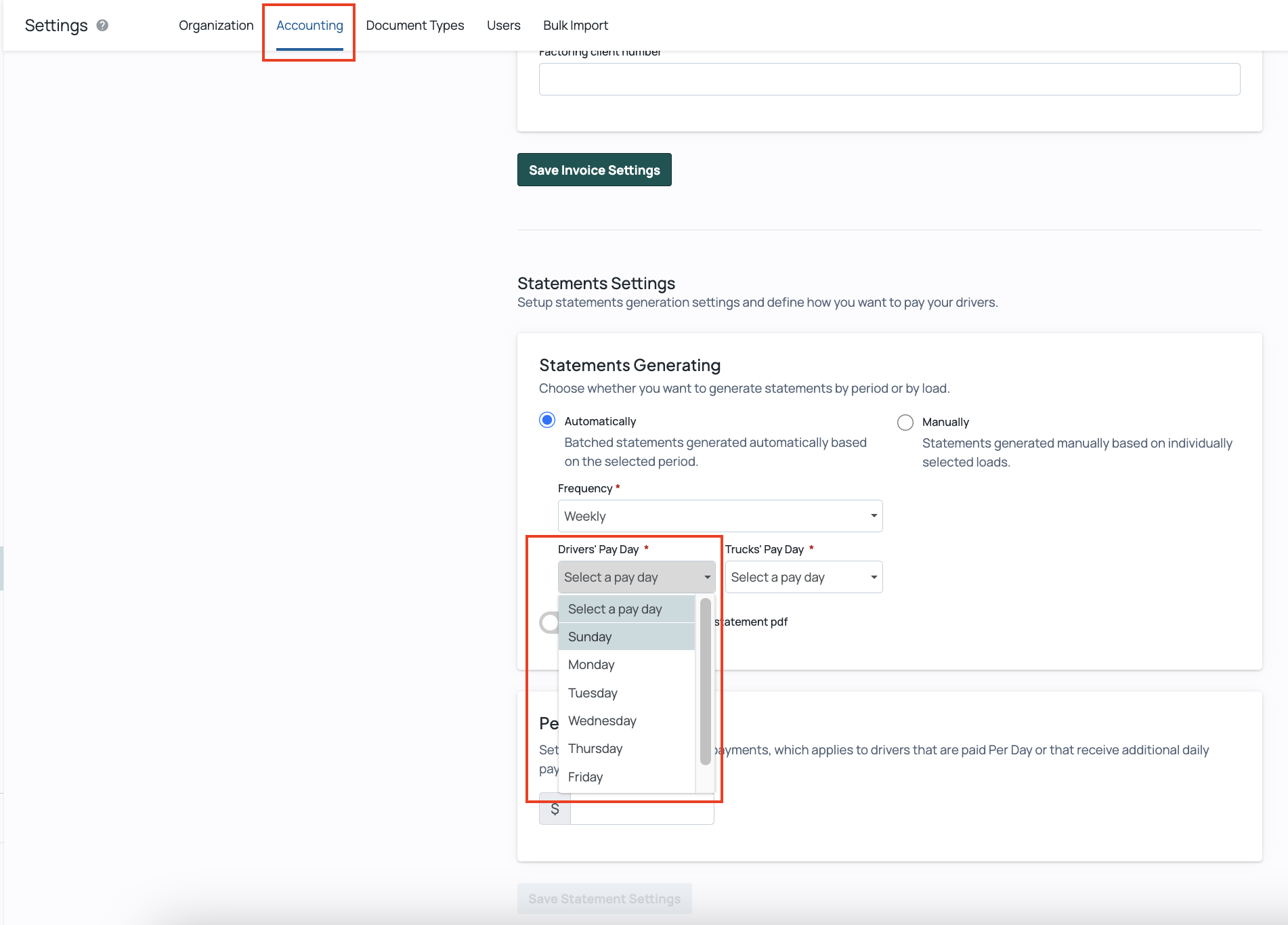# Setting Drivers' Payday for Weekly Statements

• Updated

The payday is the day of the week when drivers are paid for loads they have delivered. In Carrier TMS, the Payday setting will calculate the pay period of the driver’s statement.

For example, if the selected payday is Friday, the drivers’ statement cycle will begin on Saturday at 00:00 and end on the following Friday at 23:59.

### To select the payday:

1. On your left dashboard, go to Settings > General Settings >  Accounting

2. Under Statement Settings, select the day of the week when drivers are paid and click Save ChangesImportant: If Payday is not selected, Friday will be the default payday.

0 out of 0 found this helpful

<% if (previousArticle || nextArticle) { %>
<% if (previousArticle) { %>
<% if (previousTitle) { %>

#### <%= previousTitle %>

<% } %> <%= previousArticle.title %>
<% } %> <% if (nextArticle) { %>
<% if (nextTitle) { %>

#### <%= nextTitle %>

<% } %> <%= nextArticle.title %>
<% } %>
<% } %>
<% if (allItems.length > 1) { %>

<% } %>
<% var getColumnClasses = function(numberColumns) { var classNames = 'col-12'; if (numberColumns >= 2) classNames += ' md:col-6'; if (numberColumns >= 3) classNames += ' lg:col-4'; if (numberColumns >= 4) classNames += ' xl:col-3'; return classNames; } %>

##<%= heading %>

<% } %>
<% blocks.forEach(function(block, index) { %>
• <% if (imageHeight) { %><% } %> <% if (block.name) { %>

## <%= block.name %>

<% } %> <% if (block.description) { %>

<%= block.description %>

<% } %>
• <% }) %>

##Featured articles

<% if (articles.length) { %>
<% articles.forEach(function(article) { %>
<%= Util.getExcerpt(article.body, 0) %>
<% }); %>
<% } else { %>

empty

<% } %>
<% var getColumnClasses = function(numberColumns) { var classNames = 'col-12 mb-4'; if (numberColumns >= 2) classNames += ' md:col-6'; if (numberColumns >= 3) classNames += ' lg:col-4'; if (numberColumns >= 4) classNames += ' xl:col-3'; return classNames; } %>

##<%= heading %>

<% } %>
<% blocks.forEach(function(block, index) { %>
• <% if (imageHeight) { %><% } %>
<% if (block.name) { %>

### <% if (block.html_url) { %> <%= block.name %> <% } else { %> <%= block.name %> <% } %>

<% } %> <% if (block.description) { %>

<%= block.description %>

<% } %>
• <% }) %>

Didn't find what you were looking for?

<% if (Theme.callToActionButtons && Theme.callToActionButtons.community.length) { %> <% Theme.callToActionButtons.community.forEach(function(button) { %> <% }) %> <% } %>
<% categories.forEach(function(category, index) { %>
• <% }); %>
<% var maxSections = 5 %>
<% if (sections.length) { %>
<% sections.forEach(function(section) { %>
• <%= partial('partial-article-list-sections', { id: 'section-' + section.id, parentId: '#' + id, sections: section.sections, activeCategoryId: activeCategoryId, activeSectionId: activeSectionId, activeArticleId: activeArticleId, partial: partial }) %> <% if (section.articles.length) { %> <% } %>
• <% }); %>
<% } %>
<% if (sections.length) { %>
<% sections.slice(0, maxSections).forEach(function(section) { %>
• <%= section.name %> <%= partial('partial-section-list-sections', { parent: section, sections: section.sections, maxSections: maxSections, partial: partial }) %>
• <% }); %> <% if (sections.length > maxSections) { %>
• See more
• <% } %>
<% } %>